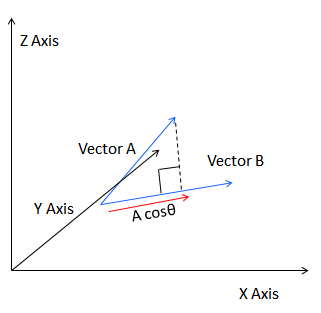# Calculate Product of Three Dimensional Vectors

Calculate the product of three dimensional vectors(3D Vectors) for entered vector coordinates.

 Vector A: Vector B:

 Scalar Product:

### The two vector A and B for dot or scalar product.

The dot product is a form of multiplication that involves two vectors having the same number of components. To determine the dot product of two vectors, we always multiply like components, and find their sum.

### Example :

Let consider value for vector A as (2 , 3 , 4) and B as (4 , 6 , 5)

The A*B = a1* b1 + a2* b2 + a3* b3

= 2x4 + 3x6 + 4x5

= 8 + 18 + 20

= 46

### Formula

The 3D vectors are using the x-y-z axes.

α is the angle between u and the x-axis.

β is the angle between u and the y-axis.

γ is the angle between u and the z-axis.

#### Therefore scalar product for unit vector:

u1 = u ⋅ i = 1 × 1 × cos α = cos α

u2 = u⋅ j = 1 × 1 × cos β = cos β

u3 = u ⋅ k = 1 × 1 × cos γ = cos γ

the unit vector u can be written as:

u = cos α i + cos β j + cos γ k